StatLect

# Distribution function

What is the probability that the realization of a random variable will be less than or equal to a certain threshold value? The distribution function of a random variable allows to answer exactly this question. Its value at a given point is equal to the probability of observing a realization of the random variable below that point or equal to that point.## Synonyms

The distribution function is also often called cumulative distribution function (abbreviated as cdf).

## Definition

The following is a formal definition.

Definition Ifis a random variable, its distribution function is a functionsuch that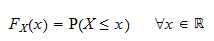where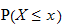is the probability thatis less than or equal to.

## Example

Suppose a random variable can take only two values (0 and 1), each with probability 1/2. Its distribution function is## Properties

Every distribution function enjoys the following four properties:

1. Increasing.is increasing, i.e.,;

2. Right-continuous.is right-continuous, i.e.,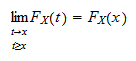for any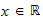;

3. Limit at minus infinity.satisfies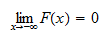4. Limit at plus infinity.satisfiesNot only any distribution function enjoys these properties, but also, for any given function enjoying these four properties, it is possible to define a random variable that has the given function as its distribution function. The practical consequence of this fact is that when you need to check whether a given function is a proper distribution function, you just need to check that it satisfies the four properties above.

## More details

More details about the distribution function can be found in the lecture entitled Random variables.

## Keep reading the glossary

Previous entry: Discrete random vector

Next entry: Estimate

The book

Most of the learning materials found on this website are now available in a traditional textbook format.

Glossary entries
Share